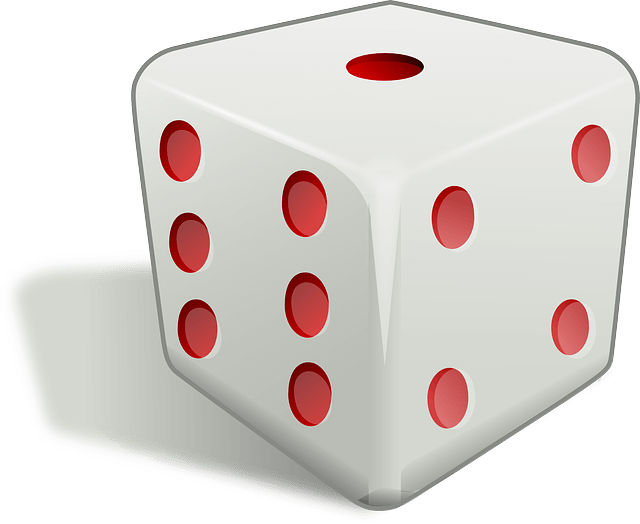# Monte Carlo Simulation

## Uncertainty

When you’re forecasting the future, you’re dealing with the unknown, or uncertainty. For simple problems, like deciding whether or not to take a bet based on the throw of a dice, it’s possible to work out the exact probability of each outcome using a simple formula. But complex, real-life decision-making problems have many variables, some fixed, some random. Arriving at a formula for forecasting the outcome of a particular decision can be impossible or impractical.

## The Monte Carlo Approach

Monte Carlo Simulation works by taking one possible solution to a complex problem and simulating it over and over again, in a multitude of different scenarios. Each simulation involves some random elements, making the outcome of each one different. After a sufficient number of simulations has been carried out, the results are aggregated into a single evaluation of the solution. The more simulations that are run, the more reliable the evaluation will be. We can run Monte Carlo Simulations on various different possible solutions, and use the resulting evaluations to compare them, and to select the best one.

## The Dice ExampleAs an example, let’s look at a problem that's deceptively simple, but which nicely illustrates uncertainty. Imagine your friend offers you a game in which you can pay him a stake for a single roll of a dice. The stake can be as low or as high as you like. If the throw of the dice is a six, he keeps your stake, otherwise he has to pay you back your stake, plus a further 26% on top. For example, if your stake is £100 and you win, you’d receive back £126, leaving you £26 better off; if you lose, then you’re £100 worse off. The problem we want to solve is: how much, if anything, should we bet?

Even though we’re only allowed one throw of the dice, once we’ve decided on our stake we can imagine the 6 possible outcomes as alternate futures. Only one of these futures can ever come to pass, but we need to consider them all when we make our bet. Five of the possible outcomes are identical, in that we win the same amount.

## Using Monte Carlo Simulation

Of course we don’t really need Monte Carlo Simulation to analyse this simple problem, but let’s see how it would work.Let’s say that we’re considering betting £100. If we randomly simulate the throw of a dice 1,000 times, probability theory would predict rolling a six around 167 times. Let’s say it actually turns out to be 164 times. In this simulation, we’d make a loss of £100 164 times, and a profit of £26 836 times. This gives an average simulated profit per throw of £5.34.

Now let’s run the Monte Carlo Simulation again with a different bet, say £200. Suppose it turns out that we roll a six 170 times. This means that we make a loss of £200 170 times, and a profit of £52 830 times. The average profit per throw is now £9.16. So, ignoring risk aversion, the Monte Carlo Simulation is telling us that a £200 bet is more profitable than a £100 bet.

## Making The Problem Harder

If we add in risk aversion, the problem becomes trickier. If we’re highly risk averse, we know that we’d simply refuse to play the game at all. If we’re only slightly risk averse, we might well be willing to make only a small bet. If we’re fairly risk tolerant, we might be willing to gamble quite a lot. If we have no risk aversion whatsoever, we might be crazy enough to bet our entire life savings.

But there's another factor too: one's current net worth. Any particular size of bet might represent a huge proportion of a poor person's net worth, but only a minute fraction of a rich person's net worth. As we can see, the perceived benefit to us of betting depends both on our risk aversion and on our net worth.

This tells us that we need to add two new variables into the problem: risk aversion and net worth. As we’ve seen, just using the average payoff won’t give us the right answer. We need to come up with a value known as a Utility for each possible bet. The Utility will be a number, but it won’t be a monetary amount. The bigger the Utility, the better the bet is for us. The actual way we calculate the Utility is central to solving this problem.

So, after incorporating these variables into our imaginary new Utility calculations, we might find that running the Monte Carlo Simulation with £1000, £10,000 and £100,000 gives us Utilities like 100, 110 and 60 respectively. In other words, there might come a point of diminishing returns. This is what we would expect for someone who has some degree of risk aversion.

We stated our problem as: how much, if anything, should we bet? By running Monte Carlo Simulations on various possible bets, we can pick the bet that gives us the highest Utility.